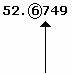## Definition Of Rounding Of Numbers

A method of approximating a number to its nearest place value is called Rounding of Numbers.

### More about Rounding of Numbers

The general method that is followed for rounding numbers or approximating numbers are:
i) Identify the last digit up to which the rounding is to be done
ii) Increase that digit by 1 if the next digit is greater than 5, or leave it the same if it is less than 5

### Examples of Rounding of Numbers

If we are supposed to round the number 3567 to the nearest hundred, then we first need to identify the digit in the hundreds place and then round the digit by increasing by 1, if the next digit is greater than 5 and put it the same if the next digit is less than 5.
3567 - The digit in the hundreds place is 5, the next digit is 6 which is greater than 5.
So, increase the digit in the hundreds place by 1, i.e. 5 + 1 = 6.
So, the rounded number is 3600.
562.54301 - If this decimal number is to be rounded to the hundredths place, then first identify the digit in the hundredths place, i.e. 4. Now check whether the digit to the right side of 4 is greater than 5 or less than 5. The digit beside 4 is 3 which is less than 5. So, don't round the number. So, the round decimal is 562.54.

### Solved Example on Rounding of Numbers

#### Ques: Round 52.6749 to the tenths place.

##### Choices:

A. 52.6
B. 52.67
C. 52.7
D. 52.68